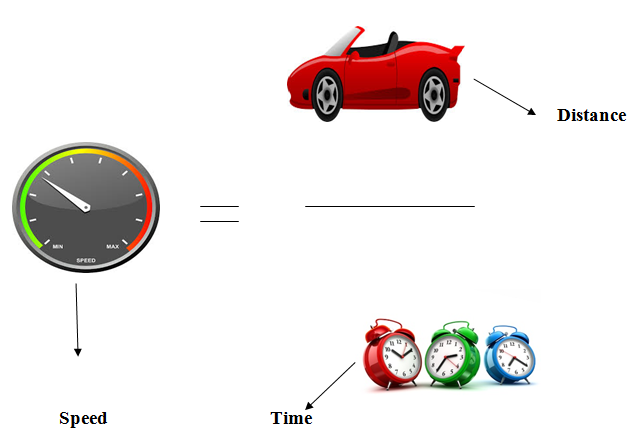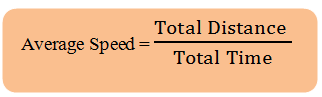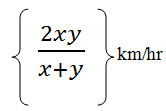# Time, Speed and Distance Made Easy

Today I am sharing techniques and concepts for Time ,speed and Distance chapter. I hope it will be beneficial to all the readers.### Conversion of  km/hr into meter/sec

Following formula is used in this case

### Conversion of meter/sec into km/h

The formula is:Some tricky questions are given below:

#### 1. Question

A Van travels 800 km in 12 hours and another 400 km in 18 hours. Find the average speed of the car.
Solution
Formula of Average speed will be apply here i.e.
Average Speed = Total Distance/Total Time
Average Speed = (800 + 400) / (12 + 18)
Average Speed = 1200/30 = 40

#### 2. Question

A horse covers a distance of 750m in 2 min 30 sec. What is the speed in km/hr of the cyclist?
Solution
Speed = 750/150 = 5m/sec
(2 min 30 seconds = 150 seconds)
Now we have to find the speed in km/hr.
5m/sec = 5 × (18/5)km/hr = 18km/hr

#### 3. Question

Find the time taken to cover a distance of 360 km by a train moving at 20 meters/second.
Solution
Time = Distance / Speed
We need to convert the distance into meter per second
so, 360  × 5/18 = 100
Now by applying the formula of Time, we get
Time = 100 / 20 = 5 hours

#### 4. Question

How many minutes does Bajira take to cover a distance of 400 m, if he runs at a speed of 20km/hr?
Solution
Bajira's speed = 20 km/hr = (20 × 5/18) m/sec = 50/9 (m/sec)
Time taken to cover 400 m = 400 × (9/50)sec = 72 sec = 1 (12/60)min = 1(1/6)min

#### 5. Question

Tata Safari covers a distance of 550 meters in 1 minute whereas a Renault Duster covers a distance of 33 km in 45 minutes. The ratio of their speeds is:
Solution
Ratio of their speeds
Speed of Tata Safari = 550/60 × 18/5 = 33 km/hr
Speed of Renault Duster = 33 / 45 × 60 = 44 km/hr
Ratio of speeds = 33 : 44
Ratio of Speeds = 3 : 4

### Calculation of Distance in case of Speed Difference

Suppose a man covers a certain distance at x km/hr and an equal distance at y km/hr. Then, to find the  average speed during the whole journey, following formula is applied.#### 6. Question

A man traveled from the village to the post - office at the rate of 25 km/h and walked back at the rate of 4 km/h. If the whole journey took 5 hours 48 minutes. Find the distance of the post - office from the village.
Solution
First of all, we have to calculate average speed by applying the formula i.e. (2xy/x+y)
Average speed = (2 ×  25 × 4) / (25 + 4)km/h = 200/29 km/h
As we know Distance = Speed × Time
Distance traveled in 5 hours 48 minutes =

Distance of Post Office from the village = 40 / 2 = 20 km#### What's trending in BankExamsToday

Smart Prep Kit for Banking Exams by Ramandeep Singh - Download here